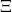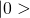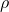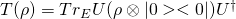## Review of R. Colbeck, R. Renner, No extension of quantum theory can have improved predictive power, Nature Communications 2:411 (2011).

#### Summary:

Colbeck and Renner (CR) inquire whether improved predictions can be achieved by any extension of quantum theory and answer this in the negative by concluding that “no extension of quantum theory can give more information about the outcomes of future measurements than quantum theory itself.” Their conclusion is the result of an analysis that is based on two stated assumptions, FR and QM: (1) Assumption FR, that measurement settings can be chosen freely, and (2) QM, that quantum theory (including measurement operations) is correct, particularly in that (a) measurement outcomes obey quantum statistics and (b) all processes within quantum theory can be considered as unitary evolution, if one takes into account the environment.

Extensions of quantum theory are described by CR as providing additional information denoted by. The proof is divided into three parts: Part I, Part II, and Part III. Part I demonstrates that assumption FR necessarily enforces thatis non-signaling. Part II demonstrates that ifis non-signaling, it cannot be used to predict the outcomes for a particular set of bipartite correlations and is thus irrelevant to quantum theory. Part III examines the case of a single measurement detector but relies explicitly on assumption (2)(b) of QM to extend the Part II result to all measurements on arbitrary pure quantum states which would establish the claim that no extension of quantum theory can have improved predictive power. The formal details of the proof are given in Supplemental Information to the paper .

#### Strengths:

The CR result is a useful demonstration of the physical consequences inherent in assumption 2(b) since Part III of their proof crucially relies on a physical implementation of this assumption. The assumption 2(b) essentially invokes Stinespring’s dilation theorem [2, p.22], which is the picture that any quantum operator can formally be written in terms of a unitary operator on a Higher Hilbert Space formed by extending the original system with an ancilla, where the ancilla is often viewed as part of the environment of the system. If the environment E is initially in the state, a unitary operator U on the composite system can always be found so that a completely positive quantum operator T() takes the following form for all,Stinespring’s dilation theorem shows that given a measurement that is characterized on average by the operator T, one can always find a unitary operator U and an additional Hilbert space such that the result of any measurement is the same, on average, as what would be found if the system interacts with an external ancilla via U. This implies that T cannot be distinguished on average from that expected under the measurement postulate.

CR are essentially able to demonstrate that if one is able to physically ignore the ancilla of assumption (2)(b), then quantum theory is complete and cannot have any physically relevant extensions.

#### Weaknesses:

Assumption (2)(b) might appear to be a tautology because of Stinespring’s theorem but its use assumes that a particular coupling occurs between system and environment in the process of invoking the measurement postulate. The devotion to describing irreversible processes in terms of reversible unitary operators of a larger system has been called, in the quantum information literature, going to The Church of the Higher Hilbert Space. However, whether or not Nature actually utilizes such particular couplings to achieve measurement is a question that can be experimentally determined.

The CR work demonstrates that as long as one is able to physically ignore the ancilla in the Stinespring dilation, then any extension of quantum theory cannot be proven either theoretically or experimentally. If this were the case, the current postulates of quantum mechanics would be complete. However, we have demonstrated in our book [3, Ch. 3] using two space-like separated detectors that it is possible in principle within the current form of quantum mechanics to experimentally detect the entanglement if the interaction is unitary and for which all parts of the system, including any ancilla and the local environment surrounding each detector are included in the description. One cannot implement an arbitrary unitary U as required under the Stinespring dilation for the case of two space-like separated detectors as direct interaction between the detectors then becomes physically prohibited due to the constraints of relativity. It should be noted that Part III of the CR proof utilized only a single detector and did not examine this type of configuration.

In more detail, we demonstrated that unitary evolution can be experimentally distinguished from measurement and developed specific unitary versus measurement discrimination tests (UMDT) using both Bell’s inequalities and Steering inequalities. One of the reasons that we constructed our UMDT was to show the incompleteness of the current postulates of quantum mechanics. The use of space-time separation in our UMDT is to show unequivocally that it is not possible that there are influences between the two detectors. That is, there are no “loopholes” that could be used to contradict the main point of the incompleteness.

These tests incorporate the distinction between what we defined [3, Ch. 4] as the physical measurement problem, which addresses the physical reasons and the conditions that measurement occurs, versus the philosopher’s measurement problem, which proposes interpretations of the current incomplete von Neumann postulates that presuppose measurement has occurred, but do not tell us the reasons nor the conditions for which measurement occurs.

In our characterization of physical measurement theories [3, Ch. 4], we also considered the possibility of a second class of theories, denoted as Category 2, which make the claim that one or more of the requirements for a theory of measurement are impossible to achieve by virtue that the conditions under which a measurement occurs prohibit one or more of the requirements from being met. In this case, there would be an in-principle failure of the ability to experimentally discriminating Schrödinger unitary from non-Schrödinger evolution. It might be further argued that the CR result is a Category 2 theory if it is claimed that it is for some fundamental reason such as inherent irreversibility to include the ancilla or environment in order to fully detect entanglement. However, even in such a case, one would need to demonstrate that a fundamental experimental limitation exists which causes irreversibility. So far, no such fundamental experimental limitations have been reported that we are aware of. And if it turned out to be correct, there would still need to be an augmentation of current quantum mechanics that establishes the conditions for which a Category 2 theory is needed, for example the specific conditions under which such irreversibility occurs.

CR’s assumption (2)(b) is simply too strong to reach the conclusion claimed in the paper’s title; it has not yet been justified by the physical evidence. Essentially (2)(b) amounts to assuming a particular type of solution to the quantum measurement problem. One cannot simply assume a solution to the quantum measurement problem to reach strong conclusions–as if they are correct: the needed coupling to the environment would need to be shown to exist and in addition to that, that there exists in-principle irreversibility or other justifiable physics that meets the requirements to establish a Category 2 theory. These are tall orders, and we believe assumption (2)(b) is nothing more than mere conjecture rather than a scientifically valid assumption. Hence, as the conclusion is based on conjecture at this time, their conclusion can only be taken as conjecture as well.

#### Conclusions:

CR demonstrate that if one is able to ignore the ancilla of assumption (2)(b) of QM, then quantum theory is complete and cannot have any physically relevant extensions.

However, when the additional environment is included, a UMDT test exists which can discriminate unitary evolution from measurement. One cannot simply ignore the additional particles required to accomplish the Stinespring dilation. Results such as CR are essentially dispersing the entanglement into the environment so that a unitary solution to the measurement problem is being forced. Even if the entanglement disperses into the environment, it still physically exists and cannot be disregarded.

Another way to view the UMDT is that the degree of superposition of a system could be considered as a hidden variable and a unitary operator derived to always give a product state result. However, one cannot encode the basis being used to measure without violating relativity for space-like separated detectors. That is, one would need to utilize an operational unitary on one device as a function of the measurement basis made on the other device. But this is impossible without violating relativity. Then by using a Bell experiment, one can eliminate the unitary solutions.

Interestingly, the second author of this paper wrote another Nature Communications paper recently (see our review), which shows a contradiction between two observers that assume closed systems obey unitary evolution, and for which each individual observer utilizes the measurement postulate when measuring. Although their results appear correct, this second paper still does not go sufficiently beyond their result for the reader to grasp all the essentials needed to fully understand the measurement problem. We recommend the aspiring measurement problem researcher that has a background in quantum information theory purchase our book , go through it in detail, watch our videos, and forward us any remaining questions, whereupon we expect that researcher will have achieved a sufficient understanding of the measurement problem to begin to conduct research. Without a sufficient in-depth understanding of this problem, we believe one is wasting time in proposing solutions.

 Supplemental Information: R. Colbeck, R. Renner, No extension of quantum theory can have improved predictive power, Nature Communications 2:411 doi: 10.1038/ncomms1416 (2011).

 J. Preskill, Lecture Notes for Ph219/CS219: Quantum Information, Chapter3, 2015.

 M. Steiner and R. Rendell, The Quantum Measurement Problem. Inspire Institute, 2018.